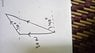# Solving vector components

• afifbaha
The magnitude of the second force is yet to be calculated, but it is certainly large. The angles in the triangle are all the same, so the triangle will be similar to a triangle that I draw with the exact same angles. If I know one side, then I use a scale, and we will get the same answer for the lengths. That's the "magic" of similar triangles.f

#### afifbaha

Member warned about posting with no template and no effort
An upward force of magnitude 30N is combined with a second force F which makes an angle of 10degree with the horizontal. The resultant makes an anglre of 40degree with the horizontal.

a. Determine the magnitude of the second force
b. Determine the magnitude of the resultant

I have no idea how to do it

IN fact if you make a good scale diagram, you could answer the question pretty closely and I think it would give you a good feel for the algebraic approach,

IN fact if you make a good scale diagram, you could answer the question pretty closely and I think it would give you a good feel for the algebraic approach,

Ok this is my diagram. Then what?

#### Attachments

•1465917259808665583887.jpg
30.7 KB · Views: 387
Well, if you made it to scale, you could just measure the lines now and that would be your answer. But if you are required to come up with an algebraic answer perhaps some trig can help you here. Can you think of some trigonometry that could help you find the sides of that shape?

Well, if you made it to scale, you could just measure the lines now and that would be your answer. But if you are required to come up with an algebraic answer perhaps some trig can help you here. Can you think of some trigonometry that could